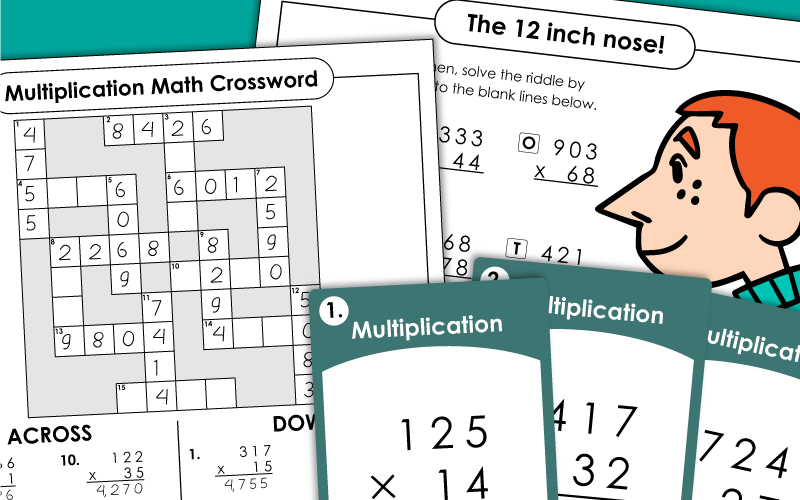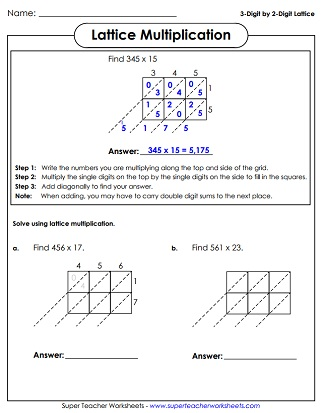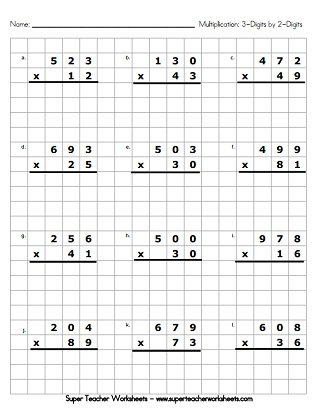# Multiplication Worksheets (3-Digits Times 2-Digits)

Worksheets to teach students to multiply pairs of 3-digit and 2-digit numbers together. There are activities with vertical problems, horizontal problems, and lattice grids. Enjoy a variety of crossword puzzles, math riddles, word problems, a Scoot game, and a custom worksheet generator tool.## 3-Digit Times 2-DigitWorksheets

This 3-digit by 2-digit multiplication worksheet has ten vertical problems and one word problem for students to solve. (example: 452 x 36)
Use this variety worksheet to help students practice multiplying 3-digit numbers by 2-digit numbers using various styles of questions.
Why can't a nose be twelve inches long? To find the answer to the riddle, solve the three-digit by two-digit multiplication problems.
Multiply to find the answers and place the products in the crossword puzzle.  (example: 766 x 11)
This page has secret code symbols that students must decipher. After using the cypher key to reveal the factors, students multiply to find the products.
Solve the 3-digit by 2-digit multiplication problems. Then glue the puzzle pieces in the correct places on the grid to reveal a pirate picture.
Word problems to practice multiplying 3-digit numbers by 2-digit numbers (example: 299 x 22)
Graph Paper Math Drills; 3-digits times 2-digits (example: 678 x 23)
Multiplication with a monster theme; 3-digits times 2-digits (example: 345 x 82)
Follow the directions to multiply 3-digit numbers by 2-digit numbers. For example: Find the product of the numbers in the circles (320 and 30)
Each problem on this worksheet has a 3-digit factor and a 2-digit factor. Students rewrite each problem vertically and solve. (example: 193 x 37)
Multiply 4-digit numbers by 2-digit numbers. (example: 2,657 x 28) Then use the products to solve the funny riddle.

## Multi-Digit MultiplicationWorksheet Generator

Multiplication Worksheet Generator

With this tool, teachers can create their own special, customized worksheets. Begin by choosing the number of digits in each factor. Choose horizontal or vertical problems. You can even customize the footer, or input word problems.

With this set of task cards, students will practice multiplying 3-digit numbers by 2-digit numbers. Use these cards for classroom scavenger hunts, for peer practice sessions, or small group instruction.

## Lattice Multiplication

This printable will help kids learn to multiply on a lattice grid. Includes an explanation box at the top, followed by eight practice grids.
Here are six blank lattice grids. Each one measures 3 boxes by 2 boxes. Pupils can use them to multiply any 3-digit number by a 2-digit number.
More Multi-Digit Multiplication

Select a topic from our huge collection of multi-digit multiplication printables.

## Worksheet ImagesMy Account
Site Information